Documentation

# procest

Estimate process model using time or frequency data

## Syntax

``sys = procest(data,type)``
``sys = procest(data,type,'InputDelay',InputDelay)``
``sys = procest(data,init_sys)``
``sys = procest(___,opt)``
``[sys,offset] = procest(___)``

## Description

example

````sys = procest(data,type)` estimates a process model, `sys`, using time or frequency-domain data, `data`. `type` defines the structure of `sys`.`sys = procest(data,type,'InputDelay',InputDelay)` specifies the input delay `InputDelay`.```

example

````sys = procest(data,init_sys)` uses the process model `init_sys` to configure the initial parameterization.```

example

````sys = procest(___,opt)` specifies additional model estimation options. Use `opt` with any of the previous syntaxes.```

example

````[sys,offset] = procest(___)` returns the estimated value of the offset in input signal. Input offset is automatically estimated when the model contains an integrator, or when you set the `InputOffset` estimation option to `'estimate'` using `procestOptions`. Use `offset` with any of the previous syntaxes.```

## Input Arguments

collapse all

Estimation data, specified as an `iddata` object containing the input and output signal values, for time-domain estimation. For frequency-domain estimation, `data` can be one of the following:

• Recorded frequency response data (`frd` or `idfrd`)

• `iddata` object with its properties specified as follows:

• `InputData` — Fourier transform of the input signal

• `OutputData` — Fourier transform of the output signal

• `Domain``'Frequency'`

`data` must have at least one input and one output.

Time-series models, which are models that contain no measured inputs, cannot be estimated using `procest`. Use `ar`, `arx`, or `armax` for time-series models instead.

Process model structure, specified for SISO models as a character vector or string representing an acronym for the model structure, such as `'P1D'` or `'P2DZ'`. The acronym is made up of:

• `P` — All `'Type'` acronyms start with this letter.

• `0`, `1`, `2`, or `3` — Number of time constants (poles) to be modeled. Possible integrations (poles in the origin) are not included in this number.

• `I` — Integration is enforced (self-regulating process).

• `D` — Time delay (dead time).

• `Z` — Extra numerator term, a zero.

• `U` — Underdamped modes (complex-valued poles) permitted. If `U` is not included in `type`, all poles must be real. The number of poles must be 2 or 3.

For MIMO models, use an `Ny`-by-`Nu` cell array of character vectors or string array, with one entry for each input-output pair. Here `Ny` is the number of inputs and `Nu` is the number of outputs.

For information regarding how `type` affects the structure of a process model, see `idproc`.

Input delays, specified as a numeric vector specifying a time delay for each input channel. Specify input delays in the time unit stored in the `TimeUnit` property.

For a system with `Nu` inputs, set `InputDelay` to an `Nu`-by-1 vector. Each entry of this vector is a numerical value that represents the input delay for the corresponding input channel. You can also set `InputDelay` to a scalar value to apply the same delay to all channels.

System for configuring initial parametrization of `sys`, specified as an `idproc` object. You obtain `init_sys` by either performing an estimation using measured data or by direct construction using `idproc`. The software uses the parameters and constraints defined in `init_sys` as the initial guess for estimating `sys`.

Use the `Structure` property of `init_sys` to configure initial guesses and constraints for Kp, Tp1, Tp2, Tp3, Tw, Zeta, Td, and Tz. For example:

• To specify an initial guess for the Tp1 parameter of `init_sys`, set `init_sys.Structure.Tp1.Value` as the initial guess.

• To specify constraints for the Tp2 parameter of `init_sys`:

• Set `init_sys.Structure.Tp2.Minimum` to the minimum Tp2 value.

• Set `init_sys.Structure.Tp2.Maximum` to the maximum Tp2 value.

• Set `init_sys.Structure.Tp2.Free` to indicate if Tp2 is a free parameter for estimation.

If `opt` is not specified, and `init_sys` was obtained by estimation, then the estimation options from `init_sys.Report.OptionsUsed` are used.

Estimation options, specified as an `procestOptions` option set. The estimation options include:

• Estimation objective

• Handling on initial conditions and disturbance component

• Numerical search method to be used in estimation

## Output Arguments

collapse all

Identified process model, returned as an `idproc` model of a structure defined by `type`.

Information about the estimation results and options used is stored in the model's `Report` property. `Report` has the following fields:

Report FieldDescription
`Status`

Summary of the model status, which indicates whether the model was created by construction or obtained by estimation.

`Method`

Estimation command used.

`InitialCondition`

Handling of initial conditions during model estimation, returned as one of the following values:

• `'zero'` — The initial conditions were set to zero.

• `'estimate'` — The initial conditions were treated as independent estimation parameters.

• `'backcast'` — The initial conditions were estimated using the best least squares fit.

This field is especially useful to view how the initial conditions were handled when the `InitialCondition` option in the estimation option set is `'auto'`.

`Fit`

Quantitative assessment of the estimation, returned as a structure. See Loss Function and Model Quality Metrics for more information on these quality metrics. The structure has the following fields:

FieldDescription
`FitPercent`

Normalized root mean squared error (NRMSE) measure of how well the response of the model fits the estimation data, expressed as a percentage.

`LossFcn`

Value of the loss function when the estimation completes.

`MSE`

Mean squared error (MSE) measure of how well the response of the model fits the estimation data.

`FPE`

Final prediction error for the model.

`AIC`

Raw Akaike Information Criteria (AIC) measure of model quality.

`AICc`

Small sample-size corrected AIC.

`nAIC`

Normalized AIC.

`BIC`

Bayesian Information Criteria (BIC).

`Parameters`

Estimated values of model parameters.

`OptionsUsed`

Option set used for estimation. If no custom options were configured, this is a set of default options. See `procestOptions` for more information.

`RandState`

State of the random number stream at the start of estimation. Empty, `[]`, if randomization was not used during estimation. For more information, see `rng` in the MATLAB® documentation.

`DataUsed`

Attributes of the data used for estimation. Structure with the following fields:

FieldDescription
`Name`

Name of the data set.

`Type`

Data type. For `idnlarx` models, this is set to `'Time domain data'`.

`Length`

Number of data samples.

`Ts`

Sample time. This is equivalent to `Data.Ts`.

`InterSample`

Input intersample behavior. One of the following values:

• `'zoh'` — Zero-order hold maintains a piecewise-constant input signal between samples.

• `'foh'` — First-order hold maintains a piecewise-linear input signal between samples.

• `'bl'` — Band-limited behavior specifies that the continuous-time input signal has zero power above the Nyquist frequency.

The value of `Intersample` has no effect on estimation results for discrete-time models.

`InputOffset`

Empty, `[]`, for nonlinear estimation methods.

`OutputOffset`

Empty, `[]`, for nonlinear estimation methods.

`Termination`

Termination conditions for the iterative search used for prediction error minimization. Structure with the following fields:

FieldDescription
`WhyStop`

Reason for terminating the numerical search.

`Iterations`

Number of search iterations performed by the estimation algorithm.

`FirstOrderOptimality`

$\infty$-norm of the gradient search vector when the search algorithm terminates.

`FcnCount`

Number of times the objective function was called.

`UpdateNorm`

Norm of the gradient search vector in the last iteration. Omitted when the search method is `'lsqnonlin'` or `'fmincon'`.

`LastImprovement`

Criterion improvement in the last iteration, expressed as a percentage. Omitted when the search method is `'lsqnonlin'` or `'fmincon'`.

`Algorithm`

Algorithm used by `'lsqnonlin'` or `'fmincon'` search method. Omitted when other search methods are used.

For estimation methods that do not require numerical search optimization, the `Termination` field is omitted.

For more information on using `Report`, see Estimation Report.

Estimated value of input offset, returned as a vector. When `data` has multiple experiments, `offset` is a matrix where each column corresponds to an experiment.

## Examples

collapse all

Obtain the measured input-output data.

```load iddemo_heatexchanger_data; data = iddata(pt,ct,Ts); data.InputName = '\Delta CTemp'; data.InputUnit = 'C'; data.OutputName = '\Delta PTemp'; data.OutputUnit = 'C'; data.TimeUnit = 'minutes';```

Estimate a first-order plus dead time process model.

```type = 'P1D'; sysP1D = procest(data,type);```

Compare the model with the data.

`compare(data,sysP1D)`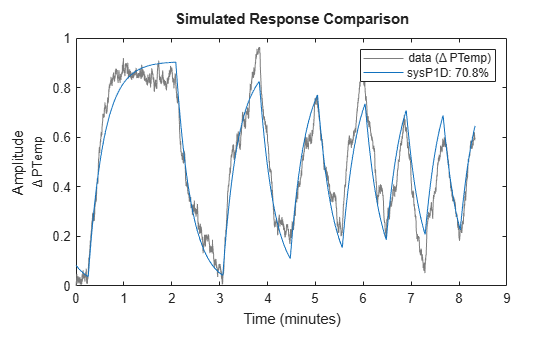Plot the model residuals.

```figure resid(data,sysP1D);```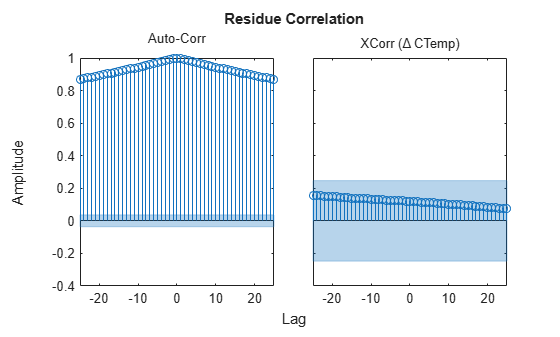The figure shows that the residuals are correlated. To account for that, add a first order ARMA disturbance component to the process model.

```opt = procestOptions('DisturbanceModel','ARMA1'); sysP1D_noise = procest(data,'p1d',opt);```

Compare the models.

`compare(data,sysP1D,sysP1D_noise)`Plot the model residuals.

```figure resid(data,sysP1D_noise);```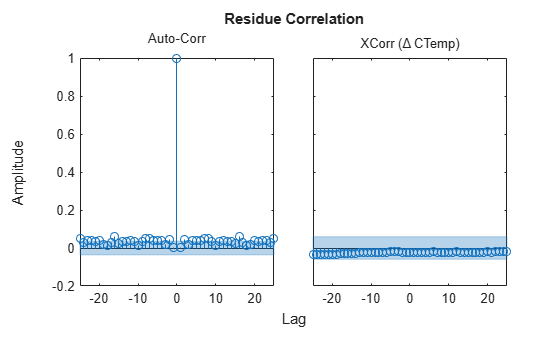The residues of `sysP1D_noise` are uncorrelated.

Use regularization to estimate parameters of an over-parameterized process model.

Assume that gain is known with a higher degree of confidence than other model parameters.

Load data.

`load iddata1 z1;`

Estimate an unregularized process model.

```m = idproc('P3UZ','K',7.5,'Tw',0.25,'Zeta',0.3,'Tp3',20,'Tz',0.02); m1 = procest(z1,m);```

Estimate a regularized process model.

```opt = procestOptions; opt.Regularization.Nominal = 'model'; opt.Regularization.R = [100;1;1;1;1]; opt.Regularization.Lambda = 0.1; m2 = procest(z1,m,opt);```

Compare the model outputs with data.

`compare(z1,m1,m2);`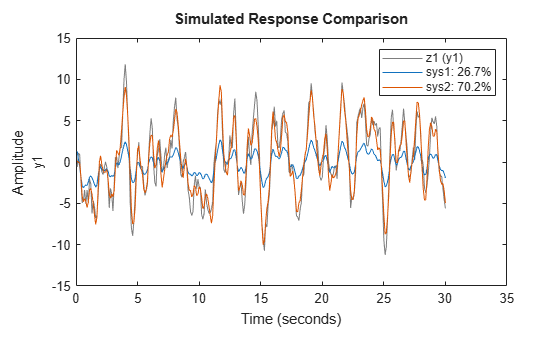Regularization helps steer the estimation process towards the correct parameter values.

Estimate a process model after specifying initial guesses for parameter values and bounding them.

Obtain input/output data.

`data = idfrd(idtf([10 2],[1 1.3 1.2],'iod',0.45),logspace(-2,2,256));`

Specify the parameters of the estimation initialization model.

```type = 'P2UZD'; init_sys = idproc(type); init_sys.Structure.Kp.Value = 1; init_sys.Structure.Tw.Value = 2; init_sys.Structure.Zeta.Value = 0.1; init_sys.Structure.Td.Value = 0; init_sys.Structure.Tz.Value = 1; init_sys.Structure.Kp.Minimum = 0.1; init_sys.Structure.Kp.Maximum = 10; init_sys.Structure.Td.Maximum = 1; init_sys.Structure.Tz.Maximum = 10;```

Specify the estimation options.

```opt = procestOptions('Display','full','InitialCondition','Zero'); opt.SearchMethod = 'lm'; opt.SearchOptions.MaxIterations = 100;```

Estimate the process model.

`sys = procest(data,init_sys,opt);`

Since the `'Display'` option is specified as `'full'`, the estimation progress is displayed in a separate Plant Identification Progress window.

Compare the data to the estimated model.

`compare(data,sys,init_sys);`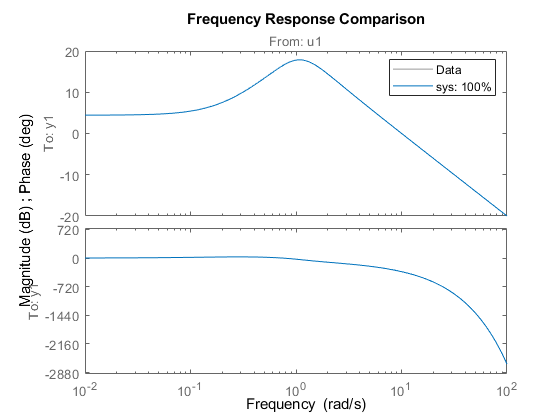Obtain input/output data.

```load iddata1 z1 load iddata2 z2 data = [z1 z2(1:300)];```

`data` is a data set with 2 inputs and 2 outputs. The first input affects only the first output. Similarly, the second input affects only the second output.

In the estimated process model, the cross terms, modeling the effect of the first input on the second output and vice versa, should be negligible. If higher orders are assigned to those dynamics, their estimations show a high level of uncertainty.

Estimate the process model.

```type = 'P2UZ'; sys = procest(data,type);```

The `type` variable denotes a model with complex-conjugate pair of poles, a zero, and a delay.

To evaluate the uncertainties, plot the frequency response.

```w = linspace(0,20*pi,100); h = bodeplot(sys,w); showConfidence(h);```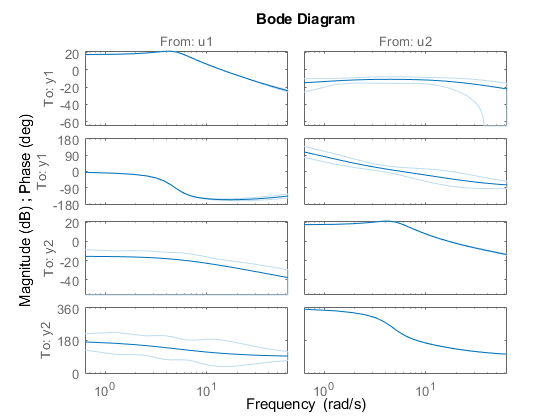```load iddata1 [sys,offset] = procest(z1,'P1DI'); offset```
```offset = 0.0412 ```

## See Also

### Topics

#### Introduced in R2012a

##### Support평가판 신청